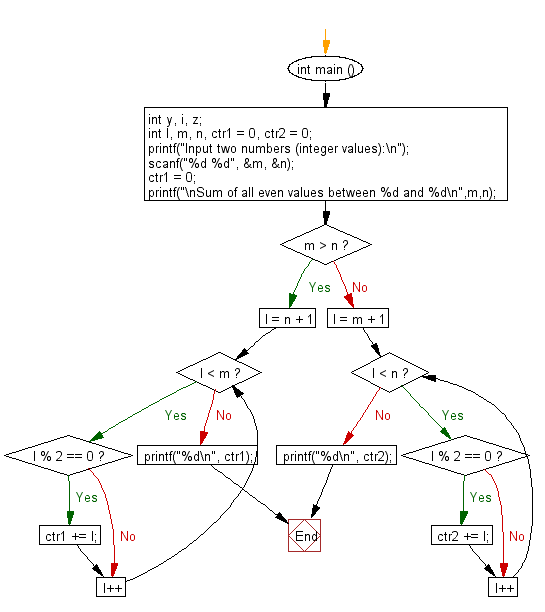﻿ C : Sum all even values between two integers, exclusive

# C Exercises: Sum of all even values between two input integers, exclusive input values

## C Basic Declarations and Expressions: Exercise-114 with Solution

Write a C program that accepts two integer values and calculates the sum of all even values between them.

Sample Solution:

C Code:

``````#include <stdio.h>

int main () {
int y, i, z;
int l, m, n, ctr1 = 0, ctr2 = 0;

// Prompt user for input
printf("Input two numbers (integer values):\n");

// Read two integer values from user and store them in 'm' and 'n'
scanf("%d %d", &m, &n);

ctr1 = 0; // Reset counter 1
printf("\nSum of all even values between %d and %d\n", m, n);

// Check if 'm' is greater than 'n'
if (m > n) {
// Loop to find and sum all even numbers between 'n' and 'm'
for (l = n + 1; l < m; l++){
if (l % 2 == 0){
ctr1 += l; // Accumulate even numbers in counter 1
}
}
printf("%d\n", ctr1); // Print the sum of even numbers
}
else {
// Loop to find and sum all even numbers between 'm' and 'n'
for (l = m + 1; l < n; l++){
if (l % 2 == 0){
ctr2 += l; // Accumulate even numbers in counter 2
}
}
printf("%d\n", ctr2); // Print the sum of even numbers
}

return 0; // End of program
}
``````

Sample Output:

```Input two numbers (integer values):
25
45

Sum of all even values between 25 and 45
350
```

Sample Output:

```Input two numbers (integer values):
27
13

Sum of all even values between 27 and 13
140
```

Flowchart:C programming Code Editor:

What is the difficulty level of this exercise?

Test your Programming skills with w3resource's quiz.

﻿